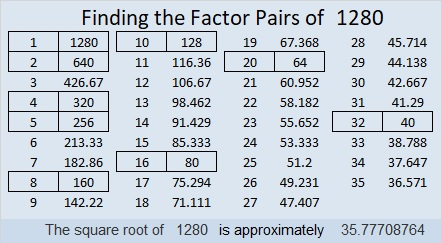# 1280 and Level 6

To me, today’s level 6 puzzle looks a little like a puppy dog. If you know or use a multiplication table, then with proper training, finding the factors of this puzzle will be no problem.Print the puzzles or type the solution in this excel file: 12 factors 1271-1280

I’d like to tell you a little about the number 1280:

• 1280 is a composite number.
• Prime factorization: 1280 = 2 × 2 × 2 × 2 × 2 × 2 × 2 × 2 × 5, which can be written 1280 = 2⁸ × 5
• The exponents in the prime factorization are 8 and 1. Adding one to each and multiplying we get (8 + 1)(1 + 1) = 9 × 2 = 18. Therefore 1280 has exactly 18 factors.
• Factors of 1280: 1, 2, 4, 5, 8, 10, 16, 20, 32, 40, 64, 80, 128, 160, 256, 320, 640, 1280
• Factor pairs: 1280 = 1 × 1280, 2 × 640, 4 × 320, 5 × 256, 8 × 160, 10 × 128, 16 × 80, 20 × 64, or 32 × 40
• Taking the factor pair with the largest square number factor, we get √1280 = (√256)(√5) = 16√5 ≈ 35.77709.1280 is the sum of the fourteen prime numbers from 61 to 127. Do you know what those prime numbers are?

32² + 16² = 1280

1280 is the hypotenuse of a Pythagorean triple:
768-1024-1280 which is (3-4-5) times 256
That triple can also be calculated from 32² – 16², 2(32)(16), 32² + 16²

Since 1280 is the 5th multiple of 256, I would expect that a number close to 1280 would be the 500th number whose square root could be simplified. That number was 1275, just five numbers ago.

This site uses Akismet to reduce spam. Learn how your comment data is processed.